#Real-Field Model: I

In this example, we will examine a model of a North Sea reservoir in detail. The model has faults, inactive cells, and disconnected components. We will show how to read, process, and visualize the model. Then we demonstrate how one can form an overlying coarse grid by partitioning the fine-grid uniformly in logical Cartesian space. We end by visualizing some of the coarse blocks and how they are connected with their neighbors.

Disclaimer: The model used in this example is unfortunately not yet publicly available.

## Read and process the model

We start by reading the model from a file in the Eclipse formate (GRDECL)

```grdecl = readGRDECL('GSmodel.grdecl');

```

The output of readGRDECL contains four fields:

• The dimension of the underlying logical Cartesian grid (keyword SPECGRID, equal 46x112x22)
• The coordinates of the pillars (keyword COORD, 6x47x113 values)
• The coordinates along the pillars (keyword ZCORN, 8x46x112x22 values)
• The flag for active/inactive cells (keyword ACTNUM, 46x112x22 values)

Since the keyword ACTNUM is present, the model is likely to contain both active and inactive cells. To be able to plot both the active and the inactive cells, we need to override the ACTNUM field when processing the input, because if not, the inactive cells will be ignored when the unstructured grid is built.

WARNING: inactive cells often contain garbage data and may generally not be inspected in this manner. Here, however, the whole formation is mostly defined in a reasonable way. By not performing basic sanity checks on the resulting grid (option 'checkgrid'=false), the graphical output may still be produced. We therefore advice that 'checkgrid' remain set in its default state of 'true'.

```actnum        = grdecl.ACTNUM;
grdecl.ACTNUM = ones(prod(grdecl.cartDims),1);
G             = processGRDECL(grdecl, 'Verbose', true, 'checkgrid', false);

```

The result of the grid processing is a new structure G, outlined below

```G   %#ok  (intentional display)

```
```G =

nodes: [1x1 struct]
cartDims: [46 112 22]
cells: [1x1 struct]
faceNodes: [1384911x1 uint32]
faces: [1x1 struct]
cellFaces: [659767x2 uint32]

```

## Inspect the whole model

Having obtained the grid in the correct unstructured format, we first plot the outline of the whole model and highlight all faults.

```newplot; subplot('position',[0.025 0.025 0.95 0.95]);
plotGrid(G,'FaceColor','none','EdgeColor',[0.65 0.65 0.65]);
plotFaces(G,find(G.faces.tag>0),'FaceColor','red');
axis equal off; view(-80,50); zoom(1.5);

```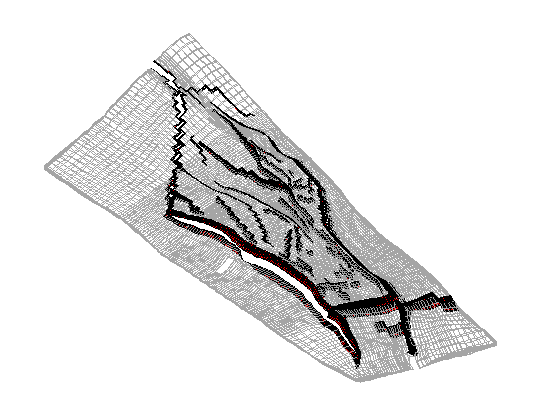Then we distinguish the active and inactive cells using the 'FaceColor' property set to 'none' for the inactive cells and to 'y' for the active cells.

```cla;
hi = plotGrid(G,find(~actnum(G.cells.indexMap)), ...
'FaceColor','none','EdgeColor',[0.65 0.65 0.65]);
ha = plotGrid(G,find( actnum(G.cells.indexMap)),'FaceColor','y');
axis equal off; view(-95,40);

```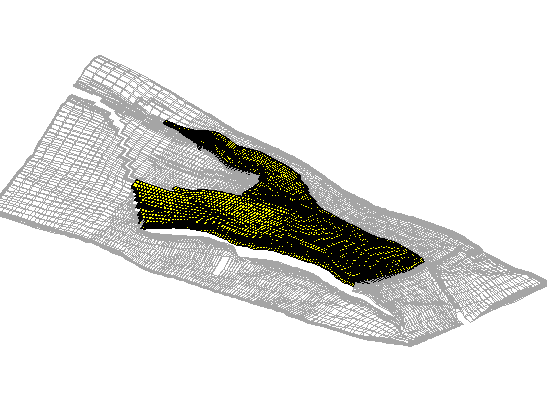## Inspect the active model

To inspect only the active model, we reset the ACTNUM field to its original values and recreate the grid. Now, inactive cells will be ignored and we therefore get a different unstructured grid.

```grdecl.ACTNUM = actnum; clear actnum;
G = processGRDECL(grdecl);
```

Here, we see that the grid has two disconnected components. Let us investigate this by first plotting the first element of the grid

```newplot; subplot('position',[0.025 0.025 0.95 0.95]);
h1 = plotGrid(G(1)); view(95,70); axis tight off;

```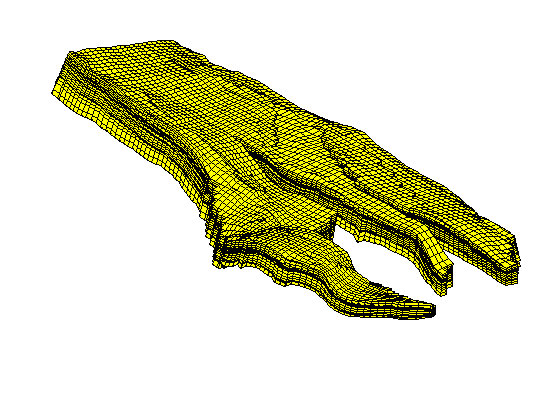Then we plot the second part of the grid in a different color and rotate the view to confirm that the two parts really are disconnected

```H2 = plotGrid(G(2),'FaceColor','r'); view(180,0);
```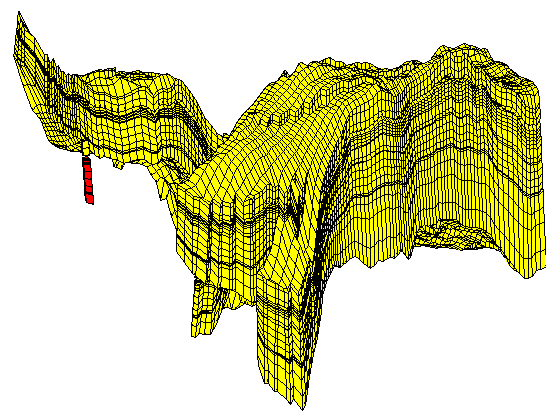## Partition the grid in logical space

From now on, we disregard the twelve disconnected cells and only consider the major part of the grid model. To construct a coarse grid, we try to partition the grid uniformly as 6x12x3 coarse blocks in index space. This will partition all cells in the logical 46x112x22 grid, including cells that are inactive. Because the coarse dimensions are not common divisors of the fine-grid dimensions, we end up with 210 rather than 216 blocks. The number of active cells within each coarse block is shown in the bar plot below.

As we can see from the bar plot, there are several coarse block that contain no active cells. We therefore postprocess the partitioning to remove blocks that contain no active cells, and then renumber the overall partitioning, giving a new total of 147 blocks.

Because the partitioning has been performed in logical index space, we have so far disregarded the fact the some of the blocks may contain disconnected cells because of erosion, faults, etc. We therefore postprocess the grid in physical space and split disconnected blocks.

```% Partition in index space
G = G(1);
blockIx = partitionUI(G,[6 12 3]); m=max(blockIx);
newplot, subplot(3,1,1)
bar(accumarray(blockIx,1)); set(gca,'XLim',[0 m]);
title('Unprocessed');

% Remove blocks contining no active cells

blockIx = compressPartition(blockIx);
subplot(3,1,2)
bar(accumarray(blockIx,1)); set(gca,'XLim',[0 m]);
title('Compressed');

% Split disconnected blocks
blockIx = processPartition(G,blockIx);
subplot(3,1,3)
bar(accumarray(blockIx,1)); set(gca,'XLim',[0 m]);
title('Processed');

assert (all(accumarray(blockIx, 1) > 0))
```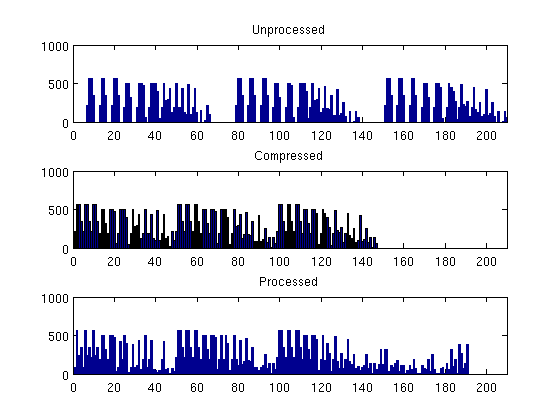We have now obtained a partitioning consisting of 191 blocks, in which each coarse block consists of a set of connected cells in the fine grid. To show the partitioning, we plot the coarse blocks using a random and cyclic color scheme for the blocks.

```newplot
subplot('position',[0.025 0.025 0.95 0.95])
blockCol = rand(max(blockIx),1)*33;
plotCellData(G,mod(blockCol(blockIx),11));
axis tight off; view(0,75); shading faceted

```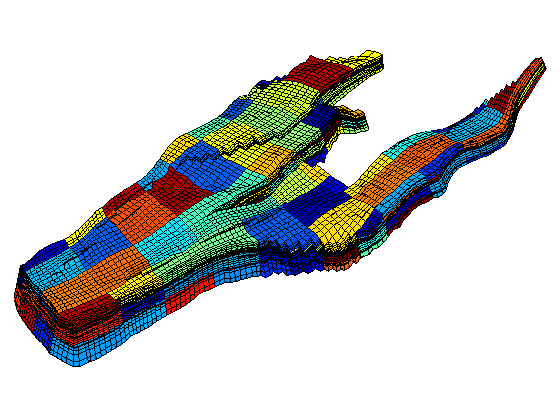From the plot above, it is not easy to see the shape of the individual coarse blocks. In the next section, we will therefore show some examples of how individual blocks can be visualized.

## Build the coarse-grid

Having obtained a partition we are satisfied with, we build the coarse-grid structure. This structure consists of three parts:

• the cell structure giving the number of blocks and the indices of the cells contained in each block
• the face structure giving the number of coarse faces and the indices of the neighbouring blocks
• a cellFaces array as in the fine-grid structure
`CG = generateCoarseGrid(G, blockIx);`

Let us now use CG to inspect some of the blocks in the coarse grid. To this end, we arbitrarily pick a few blocks and inspect these block and their neighbours. For the first block, we plot the cells and the faces that have been marked as lying on a fault

```clf; plotBlockAndNeighbors(G,CG,48); view(-90,70);
```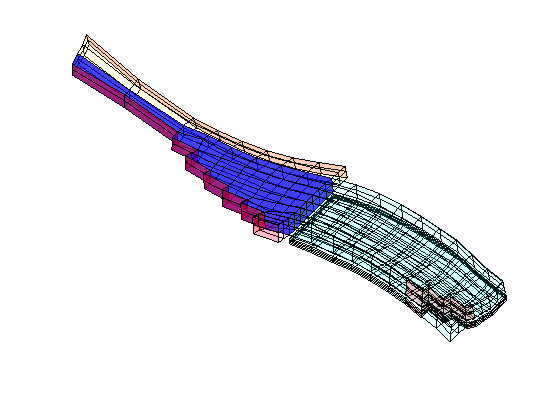For the second block, we only plot the cells and not the faulted faces

```clf; plotBlockAndNeighbors(G,CG,15,'PlotFaults',false); view(90,70);

```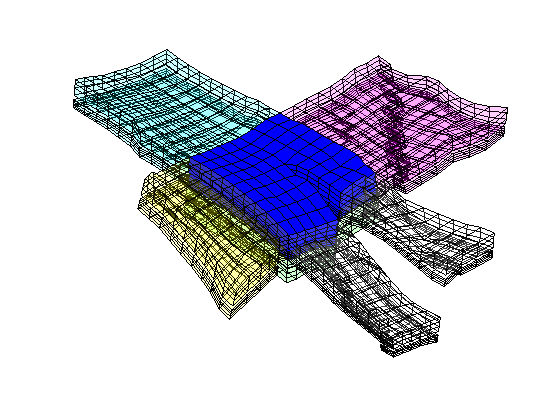The third set of neighboring blocks contains more faults

```clf; plotBlockAndNeighbors(G,CG,21); view(0,40);
```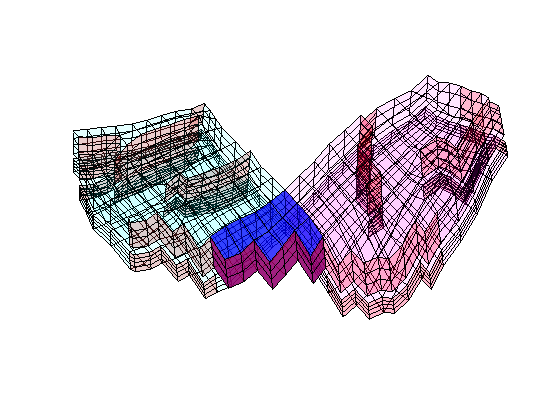We end the example by highlight six representative blocks, including the three blocks we inspected above. Notice that this way of visualization only uses the fine grid and the partition vector, and thus does not require that the coarse-grid structure has been built.

```clf
blocks = [3, 15, 21, 23, 34, 48];
col = ['b','g','r','c','m','y'];
axes('position',[0.01 0.25 0.99 0.75]);
plotGrid(G,'EdgeColor',[0.75 0.75 0.75],'FaceColor','w');
outlineCoarseGrid(G,blockIx, 'FaceColor', 'none', 'LineWidth', 2);
for i=1:6, plotGrid(G,find(blockIx==blocks(i)),'FaceColor',col(i)); end

axis tight off; view(10,90);

% Plot the chosen 6 coarse blocks
for i=1:6;
axes('position',[(i-1)/6 0.02 1/6 0.25]);
plotGrid(G,find(blockIx==blocks(i)),'FaceColor',col(i));
axis tight off, view(0,75), zoom(1.2)

end
```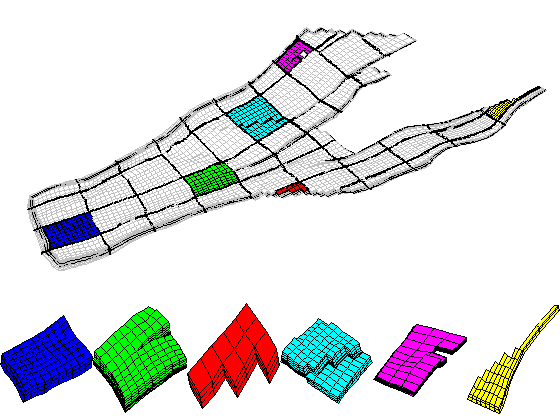Published March 31, 2009